Скачать презентацию State Preference Theory 1 Advanced economies facilitate individuals

55cf59f69312d4db5aaf31d6285786d2.ppt

• Количество слайдов: 19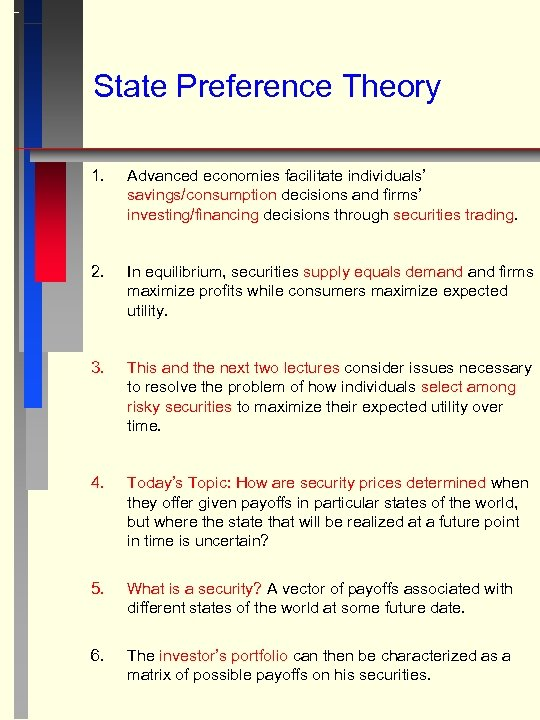State Preference Theory 1. Advanced economies facilitate individuals’ savings/consumption decisions and firms’ investing/financing decisions through securities trading. 2. In equilibrium, securities supply equals demand firms maximize profits while consumers maximize expected utility. 3. This and the next two lectures consider issues necessary to resolve the problem of how individuals select among risky securities to maximize their expected utility over time. 4. Today’s Topic: How are security prices determined when they offer given payoffs in particular states of the world, but where the state that will be realized at a future point in time is uncertain? 5. What is a security? A vector of payoffs associated with different states of the world at some future date. 6. The investor’s portfolio can then be characterized as a matrix of possible payoffs on his securities.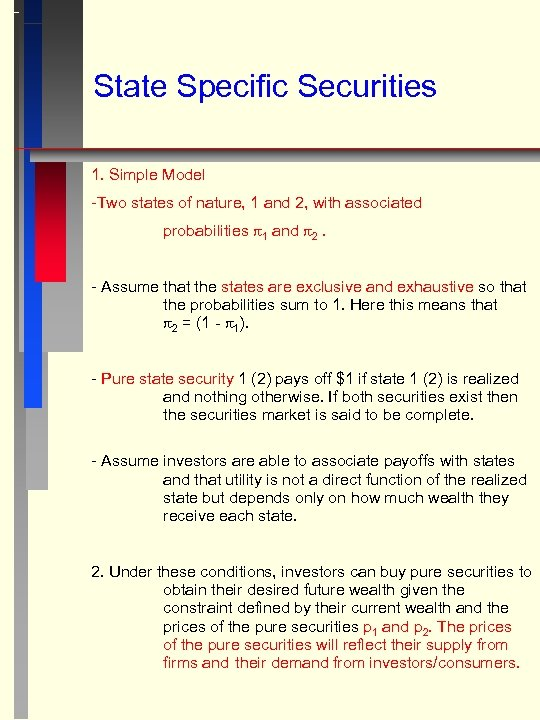State Specific Securities 1. Simple Model -Two states of nature, 1 and 2, with associated probabilities 1 and 2. - Assume that the states are exclusive and exhaustive so that the probabilities sum to 1. Here this means that 2 = (1 - 1). - Pure state security 1 (2) pays off \$1 if state 1 (2) is realized and nothing otherwise. If both securities exist then the securities market is said to be complete. - Assume investors are able to associate payoffs with states and that utility is not a direct function of the realized state but depends only on how much wealth they receive each state. 2. Under these conditions, investors can buy pure securities to obtain their desired future wealth given the constraint defined by their current wealth and the prices of the pure securities p 1 and p 2. The prices of the pure securities will reflect their supply from firms and their demand from investors/consumers.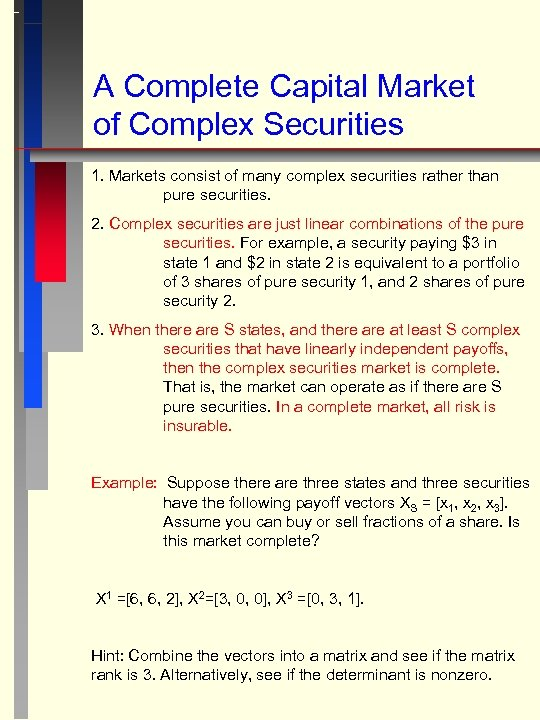A Complete Capital Market of Complex Securities 1. Markets consist of many complex securities rather than pure securities. 2. Complex securities are just linear combinations of the pure securities. For example, a security paying \$3 in state 1 and \$2 in state 2 is equivalent to a portfolio of 3 shares of pure security 1, and 2 shares of pure security 2. 3. When there are S states, and there at least S complex securities that have linearly independent payoffs, then the complex securities market is complete. That is, the market can operate as if there are S pure securities. In a complete market, all risk is insurable. Example: Suppose there are three states and three securities have the following payoff vectors XS = [x 1, x 2, x 3]. Assume you can buy or sell fractions of a share. Is this market complete? X 1 =[6, 6, 2], X 2=[3, 0, 0], X 3 =[0, 3, 1]. Hint: Combine the vectors into a matrix and see if the matrix rank is 3. Alternatively, see if the determinant is nonzero.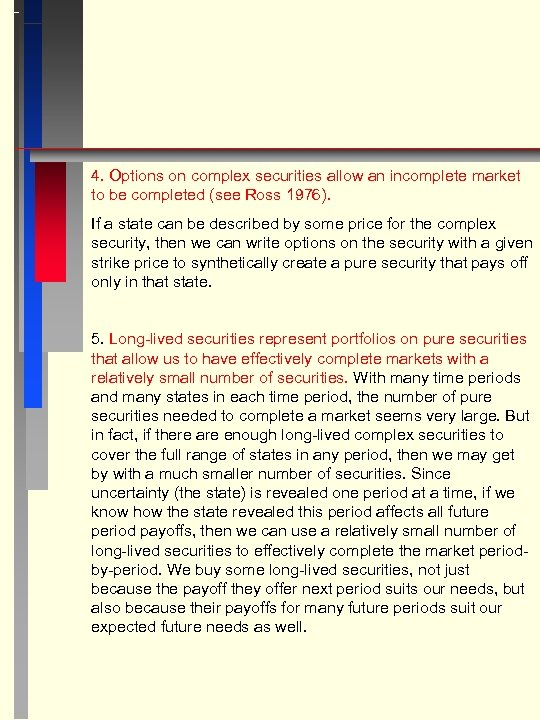4. Options on complex securities allow an incomplete market to be completed (see Ross 1976). If a state can be described by some price for the complex security, then we can write options on the security with a given strike price to synthetically create a pure security that pays off only in that state. 5. Long-lived securities represent portfolios on pure securities that allow us to have effectively complete markets with a relatively small number of securities. With many time periods and many states in each time period, the number of pure securities needed to complete a market seems very large. But in fact, if there are enough long-lived complex securities to cover the full range of states in any period, then we may get by with a much smaller number of securities. Since uncertainty (the state) is revealed one period at a time, if we know how the state revealed this period affects all future period payoffs, then we can use a relatively small number of long-lived securities to effectively complete the market periodby-period. We buy some long-lived securities, not just because the payoff they offer next period suits our needs, but also because their payoffs for many future periods suit our expected future needs as well.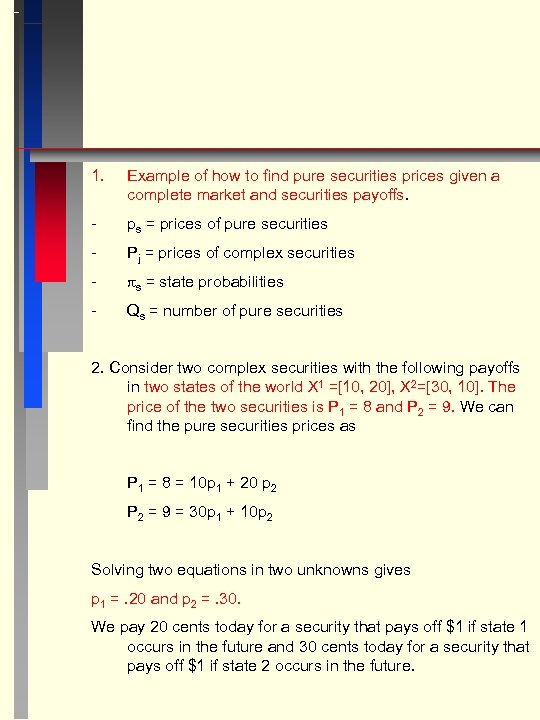1. Example of how to find pure securities prices given a complete market and securities payoffs. - ps = prices of pure securities - Pj = prices of complex securities - s = state probabilities - Qs = number of pure securities 2. Consider two complex securities with the following payoffs in two states of the world X 1 =[10, 20], X 2=[30, 10]. The price of the two securities is P 1 = 8 and P 2 = 9. We can find the pure securities prices as P 1 = 8 = 10 p 1 + 20 p 2 P 2 = 9 = 30 p 1 + 10 p 2 Solving two equations in two unknowns gives p 1 =. 20 and p 2 =. 30. We pay 20 cents today for a security that pays off \$1 if state 1 occurs in the future and 30 cents today for a security that pays off \$1 if state 2 occurs in the future.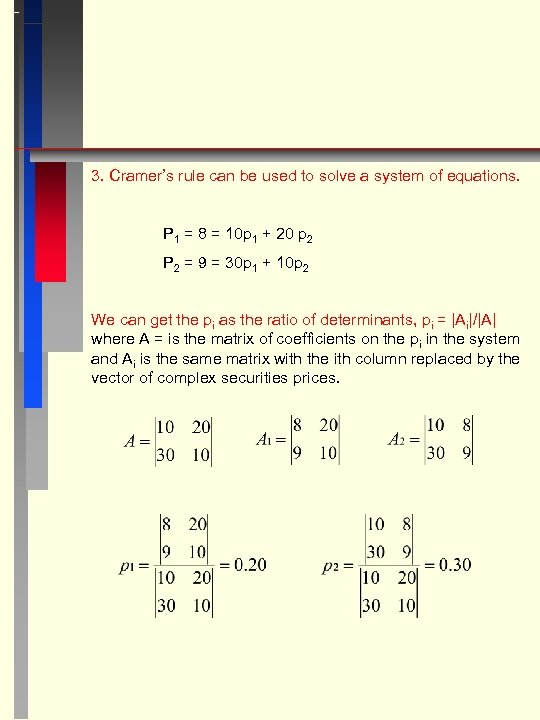3. Cramer’s rule can be used to solve a system of equations. P 1 = 8 = 10 p 1 + 20 p 2 P 2 = 9 = 30 p 1 + 10 p 2 We can get the pi as the ratio of determinants, pi = |Ai|/|A| where A = is the matrix of coefficients on the pi in the system and Ai is the same matrix with the ith column replaced by the vector of complex securities prices.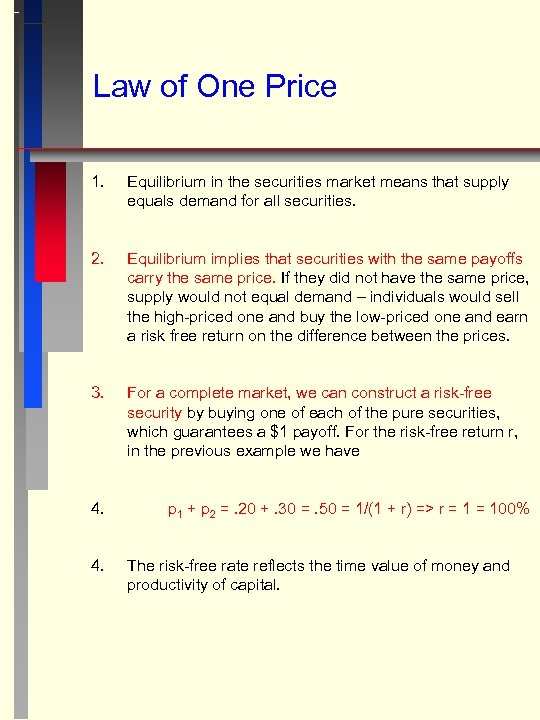Law of One Price 1. Equilibrium in the securities market means that supply equals demand for all securities. 2. Equilibrium implies that securities with the same payoffs carry the same price. If they did not have the same price, supply would not equal demand – individuals would sell the high-priced one and buy the low-priced one and earn a risk free return on the difference between the prices. 3. For a complete market, we can construct a risk-free security by buying one of each of the pure securities, which guarantees a \$1 payoff. For the risk-free return r, in the previous example we have 4. 4. p 1 + p 2 =. 20 +. 30 =. 50 = 1/(1 + r) => r = 100% The risk-free rate reflects the time value of money and productivity of capital.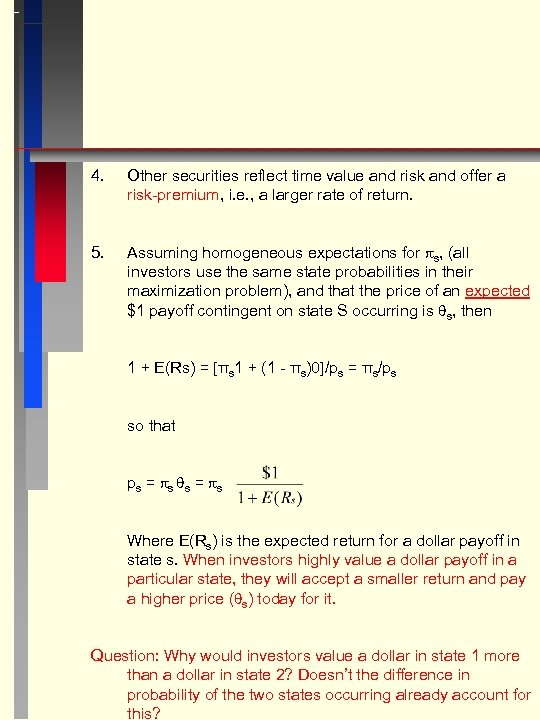4. Other securities reflect time value and risk and offer a risk-premium, i. e. , a larger rate of return. 5. Assuming homogeneous expectations for s, (all investors use the same state probabilities in their maximization problem), and that the price of an expected \$1 payoff contingent on state S occurring is s, then 1 + E(Rs) = [πs 1 + (1 - πs)0]/ps = πs/ps so that ps = s Where E(Rs) is the expected return for a dollar payoff in state s. When investors highly value a dollar payoff in a particular state, they will accept a smaller return and pay a higher price ( s) today for it. Question: Why would investors value a dollar in state 1 more than a dollar in state 2? Doesn’t the difference in probability of the two states occurring already account for this?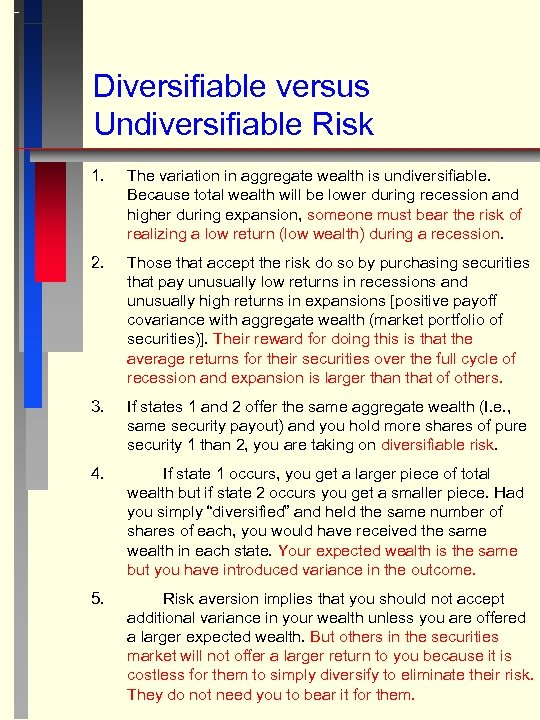Diversifiable versus Undiversifiable Risk 1. The variation in aggregate wealth is undiversifiable. Because total wealth will be lower during recession and higher during expansion, someone must bear the risk of realizing a low return (low wealth) during a recession. 2. Those that accept the risk do so by purchasing securities that pay unusually low returns in recessions and unusually high returns in expansions [positive payoff covariance with aggregate wealth (market portfolio of securities)]. Their reward for doing this is that the average returns for their securities over the full cycle of recession and expansion is larger than that of others. 3. If states 1 and 2 offer the same aggregate wealth (I. e. , same security payout) and you hold more shares of pure security 1 than 2, you are taking on diversifiable risk. 4. If state 1 occurs, you get a larger piece of total wealth but if state 2 occurs you get a smaller piece. Had you simply “diversified” and held the same number of shares of each, you would have received the same wealth in each state. Your expected wealth is the same but you have introduced variance in the outcome. 5. Risk aversion implies that you should not accept additional variance in your wealth unless you are offered a larger expected wealth. But others in the securities market will not offer a larger return to you because it is costless for them to simply diversify to eliminate their risk. They do not need you to bear it for them.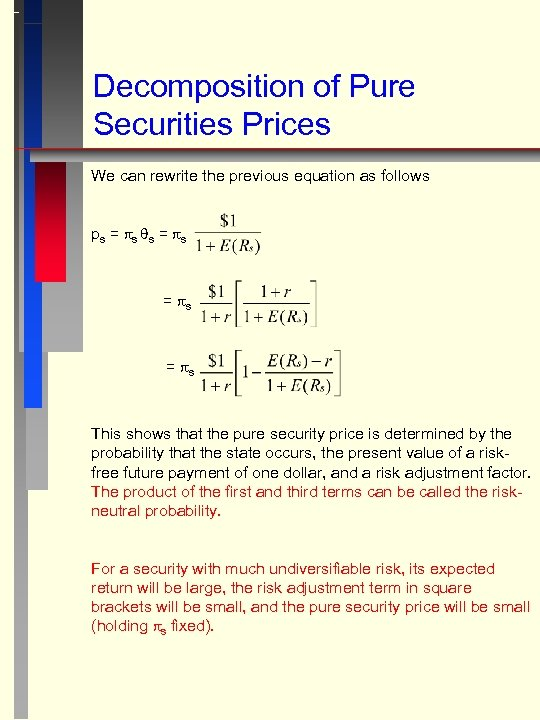Decomposition of Pure Securities Prices We can rewrite the previous equation as follows ps = s s = s = s This shows that the pure security price is determined by the probability that the state occurs, the present value of a riskfree future payment of one dollar, and a risk adjustment factor. The product of the first and third terms can be called the riskneutral probability. For a security with much undiversifiable risk, its expected return will be large, the risk adjustment term in square brackets will be small, and the pure security price will be small (holding s fixed).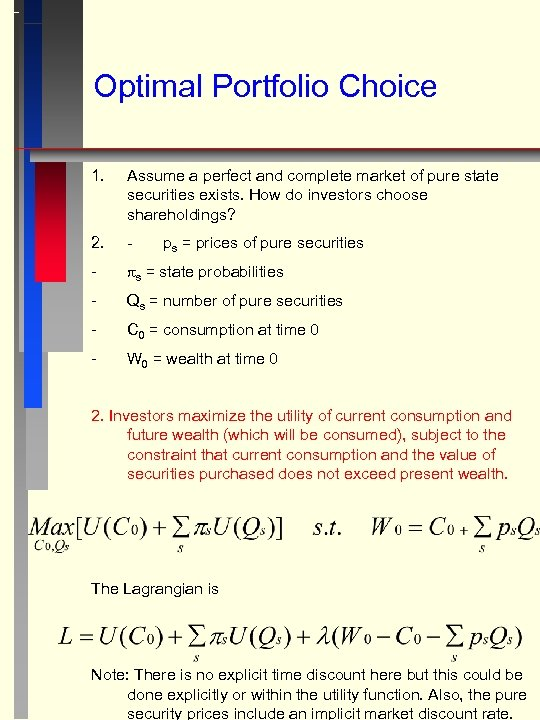Optimal Portfolio Choice 1. Assume a perfect and complete market of pure state securities exists. How do investors choose shareholdings? 2. - - s = state probabilities - Qs = number of pure securities - C 0 = consumption at time 0 - W 0 = wealth at time 0 ps = prices of pure securities 2. Investors maximize the utility of current consumption and future wealth (which will be consumed), subject to the constraint that current consumption and the value of securities purchased does not exceed present wealth. The Lagrangian is Note: There is no explicit time discount here but this could be done explicitly or within the utility function. Also, the pure security prices include an implicit market discount rate.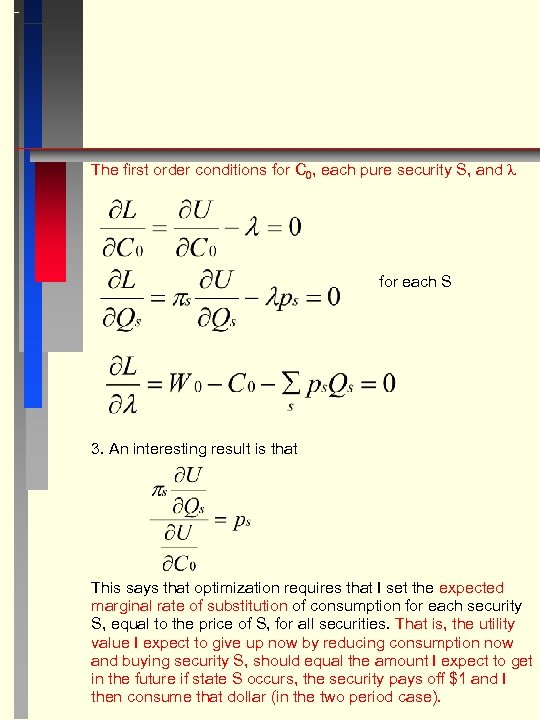The first order conditions for C 0, each pure security S, and for each S 3. An interesting result is that This says that optimization requires that I set the expected marginal rate of substitution of consumption for each security S, equal to the price of S, for all securities. That is, the utility value I expect to give up now by reducing consumption now and buying security S, should equal the amount I expect to get in the future if state S occurs, the security pays off \$1 and I then consume that dollar (in the two period case).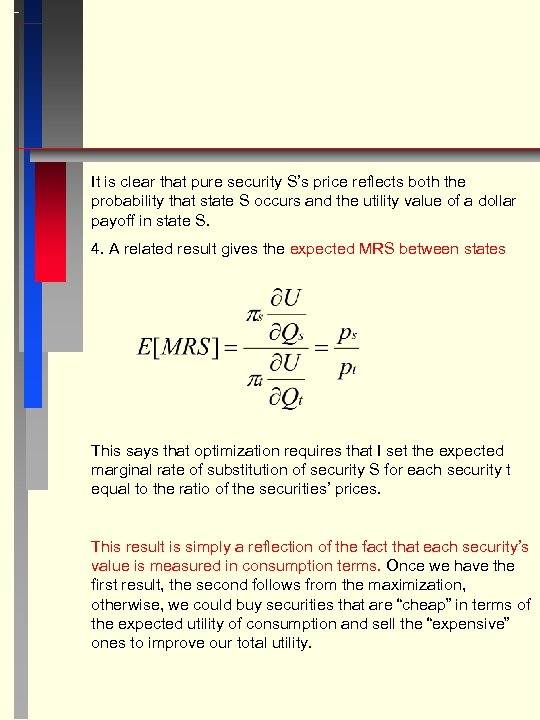It is clear that pure security S’s price reflects both the probability that state S occurs and the utility value of a dollar payoff in state S. 4. A related result gives the expected MRS between states This says that optimization requires that I set the expected marginal rate of substitution of security S for each security t equal to the ratio of the securities’ prices. This result is simply a reflection of the fact that each security’s value is measured in consumption terms. Once we have the first result, the second follows from the maximization, otherwise, we could buy securities that are “cheap” in terms of the expected utility of consumption and sell the “expensive” ones to improve our total utility.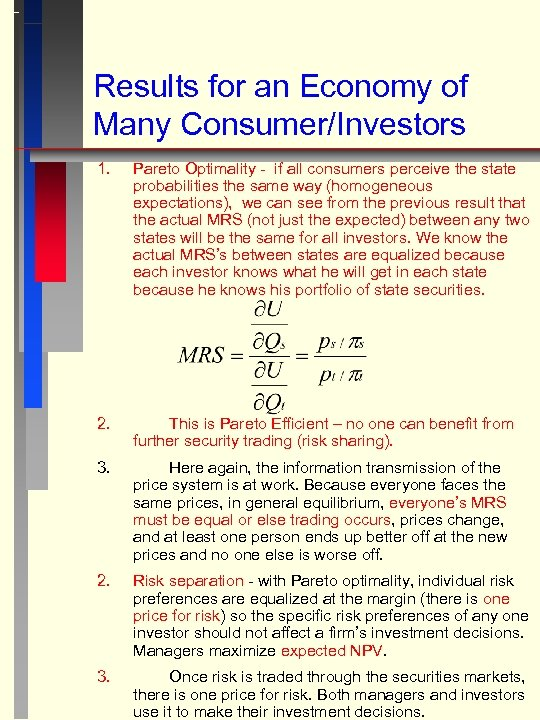Results for an Economy of Many Consumer/Investors 1. Pareto Optimality - if all consumers perceive the state probabilities the same way (homogeneous expectations), we can see from the previous result that the actual MRS (not just the expected) between any two states will be the same for all investors. We know the actual MRS’s between states are equalized because each investor knows what he will get in each state because he knows his portfolio of state securities. 2. This is Pareto Efficient – no one can benefit from further security trading (risk sharing). 3. Here again, the information transmission of the price system is at work. Because everyone faces the same prices, in general equilibrium, everyone’s MRS must be equal or else trading occurs, prices change, and at least one person ends up better off at the new prices and no one else is worse off. 2. Risk separation - with Pareto optimality, individual risk preferences are equalized at the margin (there is one price for risk) so the specific risk preferences of any one investor should not affect a firm’s investment decisions. Managers maximize expected NPV. 3. Once risk is traded through the securities markets, there is one price for risk. Both managers and investors use it to make their investment decisions.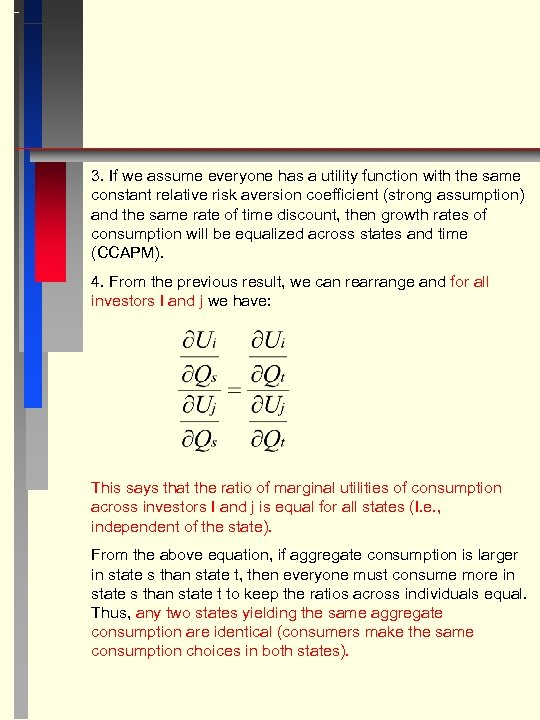3. If we assume everyone has a utility function with the same constant relative risk aversion coefficient (strong assumption) and the same rate of time discount, then growth rates of consumption will be equalized across states and time (CCAPM). 4. From the previous result, we can rearrange and for all investors I and j we have: This says that the ratio of marginal utilities of consumption across investors I and j is equal for all states (I. e. , independent of the state). From the above equation, if aggregate consumption is larger in state s than state t, then everyone must consume more in state s than state t to keep the ratios across individuals equal. Thus, any two states yielding the same aggregate consumption are identical (consumers make the same consumption choices in both states).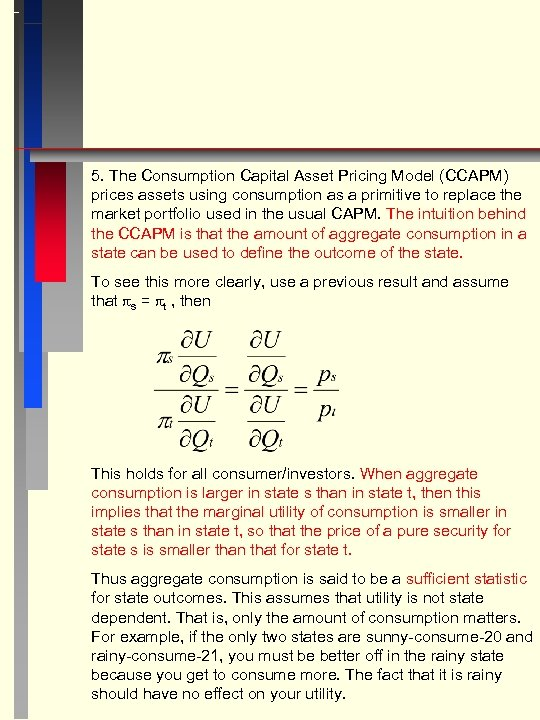5. The Consumption Capital Asset Pricing Model (CCAPM) prices assets using consumption as a primitive to replace the market portfolio used in the usual CAPM. The intuition behind the CCAPM is that the amount of aggregate consumption in a state can be used to define the outcome of the state. To see this more clearly, use a previous result and assume that s = t , then This holds for all consumer/investors. When aggregate consumption is larger in state s than in state t, then this implies that the marginal utility of consumption is smaller in state s than in state t, so that the price of a pure security for state s is smaller than that for state t. Thus aggregate consumption is said to be a sufficient statistic for state outcomes. This assumes that utility is not state dependent. That is, only the amount of consumption matters. For example, if the only two states are sunny-consume-20 and rainy-consume-21, you must be better off in the rainy state because you get to consume more. The fact that it is rainy should have no effect on your utility.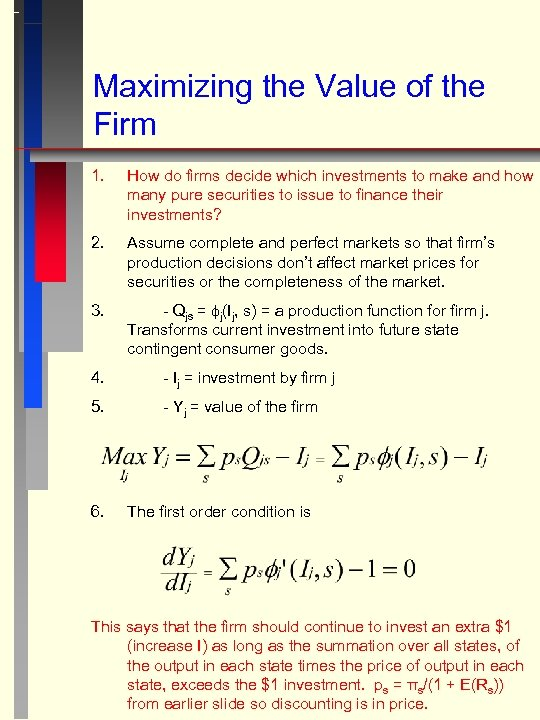Maximizing the Value of the Firm 1. How do firms decide which investments to make and how many pure securities to issue to finance their investments? 2. Assume complete and perfect markets so that firm’s production decisions don’t affect market prices for securities or the completeness of the market. 3. - Qjs = j(Ij, s) = a production function for firm j. Transforms current investment into future state contingent consumer goods. 4. - Ij = investment by firm j 5. - Yj = value of the firm 6. The first order condition is This says that the firm should continue to invest an extra \$1 (increase I) as long as the summation over all states, of the output in each state times the price of output in each state, exceeds the \$1 investment. ps = πs/(1 + E(Rs)) from earlier slide so discounting is in price.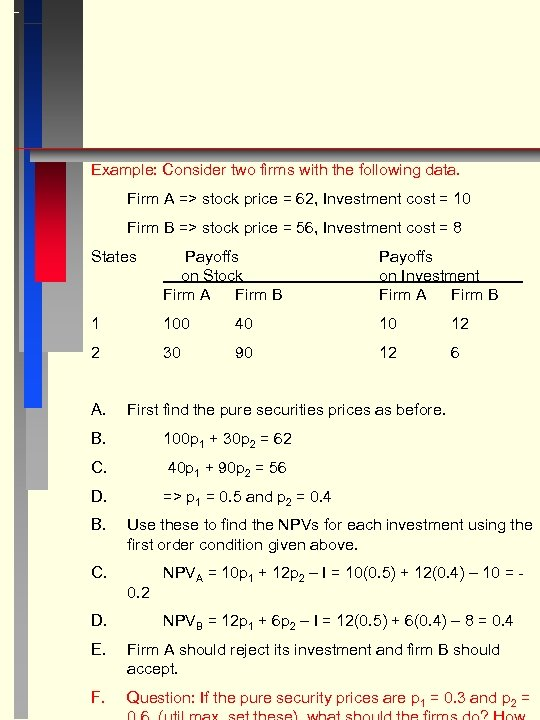Example: Consider two firms with the following data. Firm A => stock price = 62, Investment cost = 10 Firm B => stock price = 56, Investment cost = 8 States Payoffs on Stock Firm A Firm B Payoffs on Investment Firm A Firm B 1 100 40 10 12 2 30 90 12 6 A. First find the pure securities prices as before. B. 100 p 1 + 30 p 2 = 62 C. 40 p 1 + 90 p 2 = 56 D. => p 1 = 0. 5 and p 2 = 0. 4 B. Use these to find the NPVs for each investment using the first order condition given above. C. 0. 2 D. NPVA = 10 p 1 + 12 p 2 – I = 10(0. 5) + 12(0. 4) – 10 = NPVB = 12 p 1 + 6 p 2 – I = 12(0. 5) + 6(0. 4) – 8 = 0. 4 E. Firm A should reject its investment and firm B should accept. F. Question: If the pure security prices are p 1 = 0. 3 and p 2 =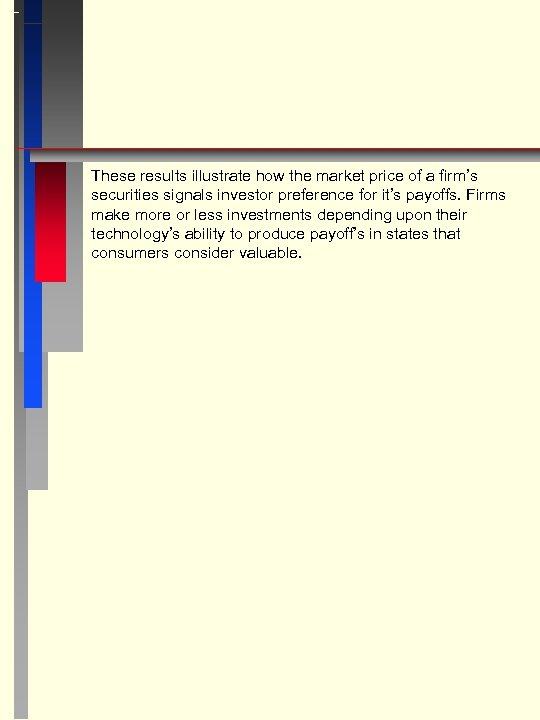These results illustrate how the market price of a firm’s securities signals investor preference for it’s payoffs. Firms make more or less investments depending upon their technology’s ability to produce payoff’s in states that consumers consider valuable.